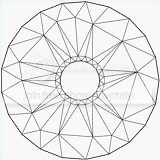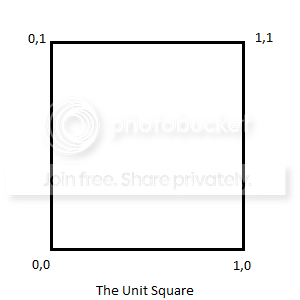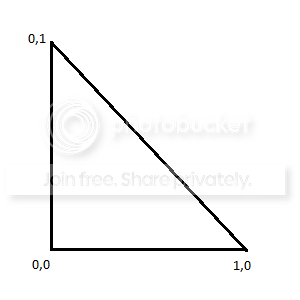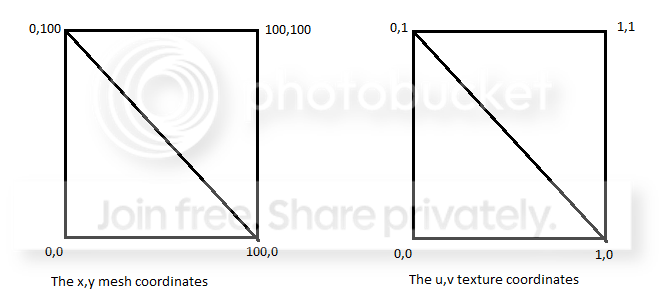# Core / mesha

Mesh Tutorial

In this tutorial, I am going to talk about what meshes are, why you might want to use them, and how to use them in Codea. To complete this tutorial, you probably want to have a basic understanding of Codea and the LUA language. Let's call the level of difficulty "intermediate."

What Meshes Are

First off, let's talk about what a mesh is. You might think of a mesh as a 3D object, and meshes ARE a basic part of 3D objects. But a mesh could more accurately be defined as as a list of polygons, and more specifically, in the case of Codea, it is a list of triangles. These lists of polygons are very useful for both 2d and 3d game creation.

Basically any 2d or 3d shape can be broken up into a bunch of triangles. Generally, the more polygons used, the smoother the shape.In modern 3d games, the models are composed of thousands (or tens of thousands) of polygons. To accomplish this feat, the iPad graphics hardware has been designed to manipulate and draw triangles extremely fast, much faster than just drawing pixels to the screen. By using meshes in Codea rather than using the sprite command, you can often drastically speed up the rendering in your game.

To create a mesh you simply run the built in mesh function, which returns an empty mesh.

```myMesh = mesh()
```

That mesh is pretty worthless without any triangles, so let's add a triangle to it. Here is a diagram of the first mesh we are going to create:And here is the code to add that triangle to our mesh:

```myMesh.vertices = {vec2(0,0),vec2(100,0),vec2(0,100)}
```

And now we have a functional mesh. Let's make a quick program to test it out:

```function setup()
myMesh = mesh()
myMesh.vertices = {vec2(0,0),vec2(100,0),vec2(0,100)}
end

function draw()
background(40, 40, 50)
myMesh:draw()
end
```

Run this and you will see our grey triangle appear in the bottom left hand corner of the screen at the origin. The triangle is rendered in the current global fill color (default is grey) because we have not set vertex colors. Let's try giving it color first, and we will graduate to textures later.

Coloring Meshes

To add color to a mesh you pass a list of colors to the mesh that is equal in length to the number of vertices. This means that for every vertex in the list, you must provide a color. Our mesh currently has 1 triangle and therefore 3 vertices, so we need to give the mesh 3 colors. Here is the updated program with colors added:

```function setup()
red = color(255, 0, 0, 255)
green = color(0, 255, 0, 255)
blue = color(0, 0, 255, 255)

myMesh = mesh()
myMesh.vertices = {vec2(0,0),vec2(100,0),vec2(0,100)}
myMesh.colors = {red, green, blue}
end

function draw()
background(40, 40, 50)
myMesh:draw()
end
```

Now our mesh is rendered in color, and we get a pretty neat gradient effect as the colors are blended between the vertices. You can use this technique to create gradient backgrounds, buttons, etc.

Texturing Meshes

Texturing meshes works a lot like coloring meshes, but instead of passing a list of colors, we will pass a list of texture coordinates. This is a process called U,V mapping. The x,y coordinate of each vertex is mapped to a coordinate on the texture, which is called the u,v coordinate, since x and y are already in use. (Hence the name U,V mapping) With the U,V coordinates, we use a "unit-square" system, which allows us to specify what portion of the texture we want to use for the triangle, instead of the exact pixels. (Don't worry, this will be explained more clearly as you follow this example.)

This is a diagram of the unit square:If you want to use the full width of the image, you would use 1 as the width. To use half of the width, you would use .50 as the width. Let's take an image from the Codea spritepacks and use it as our texture. First get rid of the color code as we are now texturing instead. Next we want to make a triangle in U,V coordinates that matches our mesh triangle. It will look something like this:So the texture coordinates for our triangle are going to be: `0,0 1,0 0,1` Can you see how those relate to our vertices? Remember they are: `0,0 100,0 0,100`

Here is the updated code:

```function setup()
img = readImage("Planet Cute:Icon") --Get the image

myMesh = mesh()
myMesh.vertices = {vec2(0,0),vec2(100,0),vec2(0,100)}
myMesh.texture = img --Set the image as texture
myMesh.texCoords = {vec2(0,0),vec2(1,0),vec2(0,1)}
end

function draw()
background(40, 40, 50)
myMesh:draw()
end
```

And if done correctly, we should now have half of the image drawn on our triangle. To see the rest of this image, I would really like to have another triangle to finish the rectangle. So, looking at this image:You should see that the triangle we need to add to our mesh has the vertices: `0,100 100,100 100,0`

And the texture coordinates for that new triangle will be: `0,1 1,1 1,0`

Lets add them to our code:

```function setup()
img = readImage("Planet Cute:Icon") --Get the image

myMesh = mesh()
myMesh.vertices = {vec2(0,0),vec2(100,0),vec2(0,100),vec2(0,100),vec2(100,100),vec2(100,0)}
myMesh.texture = img --Set the image as texture
myMesh.texCoords = {vec2(0,0),vec2(1,0),vec2(0,1),vec2(0,1),vec2(1,1),vec2(1,0)}
end

function draw()
background(40, 40, 50)
myMesh:draw()
end
```

And Voila, we have our image drawn correctly to a mesh. Now that we have learned how to draw a rectangular texture onto a mesh the hard way, lets look at a slightly simplified way of completing that same task.

The Rectangle Functions

It is very common to want to use rectangles in your meshes like we did above. To make it easier on us, Codea has a mesh function called addRect. addRect automatically adds two right(angle) triangles to your mesh that together make a rectangle. It can also automatically create the proper texture coordinates. To replicate the work we did above, we could use:

```myMesh:addRect(50,50,100,100)
```

Much easier, huh? The reason we used 50,50 there instead of 0,0 is because the addRect function creates the mesh CENTERED on the x,y coordinate given.

Note: Using the addRect function after passing in a list of vertices can cause some of the original triangles to be overwritten. It is a good practice to only use one method of creating triangles and not mix the two.

So the following code could do what we did above:

```function setup()
myMesh = mesh()
myMesh.texture = img
end

function draw()
background(40, 40, 50)
myMesh:draw()
end
```

You can also adjust the texture on rectangles. This is especially useful if you want a mesh with one texture stretched over a grid of rectangles. When you create a new rectangle using addRect, the function will return a unique identifier for that rectangle that can be used to modify the texturing (u,v mapping) of the rectangle. You do it like this:

```idx = myMesh:addRect(50,50,100,100)
myMesh:setRectTex(idx, 0, 0, 1, 1)
```

The arguments you pass to the setRectTex function are: (index, u, v, width, height)

So if I want to only use the bottom-left 1/4th of the image as my texture I would do so like this:

```myMesh:setRectTex(idx,0,0,.50,.50)
```

And if I wanted to use the top left 1/4 of the image I would use:

```myMesh:setRectTex(idx,0,.50,.50,.50)
```

When creating rectangular meshes, it is generally much easier to use the rectangle functions. If you are just using meshes to draw sprites quickly on the screen, then using addRect will likely be much more user-friendly than creating a list of vertices and creating a complimentary texture coordinate list.

Homework

Now that you have the idea of how to create meshes, you should try putting your knowledge into action. Try completing one of these challenges on your own:

1. Try adding color AND texture to the same mesh. You can color and shade images this way
2. Create a mesh with a grid of 9 rectangles. Use setRectTex to make one image cover the entire grid.
3. Create an animation by changing the texture of the mesh in a loop
4. Create a shape other than a rectangle by passing in a list of vertices. You may need to draw it out on paper first to figure out the coordinates.

Updated